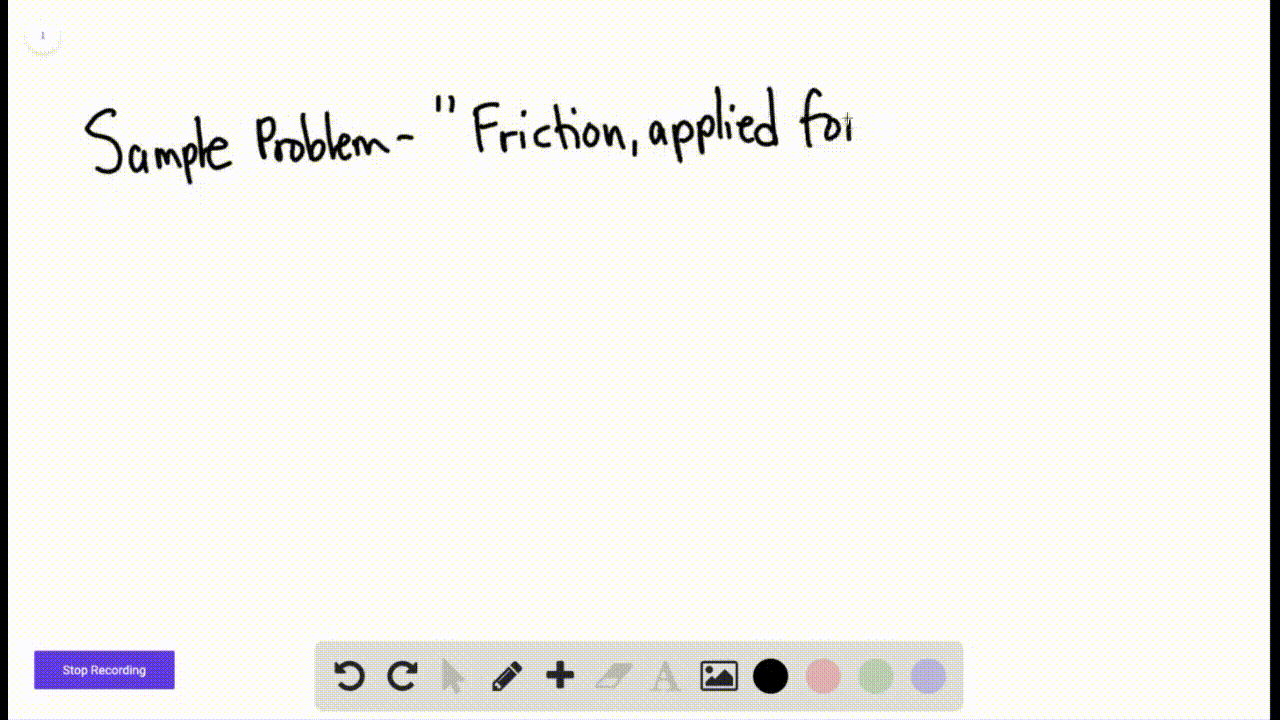Sign up for our free STEM online summer camps starting June 1st!View Summer Courses### Figure $6-22$ shows the cross section of a road c…

04:42
AH
Carnegie Mellon University

Need more help? Fill out this quick form to get professional live tutoring.

Get live tutoring
Problem 13

A worker pushes horizontally on a 35 $\mathrm{kg}$ crate with a force of magnitude 110 $\mathrm{N}$ . The coefficient of static friction between the crate and the floor is 0.37 (a) What is the value of $f_{x, \max }$ under the circumstances? (b) Does the crate move? (c) What is the frictional force on the crate from the floor? (d) Suppose, next, that a second worker pulls directly upward on the crate to help out.
What is the least vertical pull that will allow the first worker's 110 $\mathrm{N}$ push to move the crate? (e) If, instead, the second worker pulls horizontally to help out, what is the least pull that will get the crate moving?

(a) $f_{s, \max }=126.9 \mathrm{N}$
(b) $\mathrm{No}$
(c) $f_{s}=-110 \mathrm{N}$
(d) 46 $\mathrm{N}$
(e) 17 $\mathrm{N}$

## Discussion

You must be signed in to discuss.

## Video Transcript

So we're going to Dino to the force exerted by the worker on the create as f equaling 110 Newtons. And we can say that then the maximum static frictional force would be equaling the coefficient of static friction multiplied by the force normal for party. When we apply Newton's second Law here in the vertical direction, we can say that the force normal would be equal to the gravitational force mg. And so we can say that the maximum static frictional force would then be equaling two the coefficient of static friction mg. This is gonna be equaling 2.37 multiplied by 35 kilograms multiplied by the acceleration due to gravity 9.8 meters per second squared. And we find that then this is equaling approximately 130 Newton's, and we can then say that the maximum static frictional force is greater than F for part B. We can see that the block is initially at rest and it stays at rest because the force F is less than the maximum static fictional force. If the force can't exceed that maximum static fictional force than the block will stay at rest and we can say en Bloc stays at rest. And for part C, we can say that applying Newton's second law here to the horizontal direction, we can say that the magnitude of the static fictional force exerted here on the create would be equaling f equaling 110 Newtons. So I'll be your answer for part c and then for part D. We have that first, we're going to say, Ah, we're going to see the upward force exerted by the second worker as f sub too exerted why Second worker and given this weekend, apply again Newton's second law. We can then see that the normal force here is gonna be equaling the gravitational force mg minus the magnitude efs up to. And this leads to the maximum static frictional force equaling the coefficient of static friction multiplied by M G minus F sub too. So, in order to move the create, the force F from the previous worker has to be greater than the maximum static frictional force. And this is equaling music s mg minus except two. And so we can say 110 Newtons greater than 0.37 multiplied by 35 kilograms, 9 20 meters per second. Squared minus have some too. And so we can say that the minimum value that satisfies this inequality is a slightly larger value than 45.7. So we're just gonna round up and we're gonna say F sub two men rounded to two significant figures is 46 Newton's. So I'd be for Part D and then Part E. We have this final case here. Moving the crate requires a greater horizontal push from the worker than static friction. So here we can say that applying Newton's second law plus F stop too, must be greater than being maximum static frictional force. And so we can then say that 110 Newtons plus absent, too, must be greater than approximately 126.9. Newton's after plugging in our variables, and we see that of sub two men here is gonna be equaling 2 17 Newton's so I'll be your answer for party. That is the end of the solution. Thank you for watching